• 非径向方向距离函数
千次阅读 多人点赞
2020-07-08 15:05:55

点赞发Nature
关注中Science

上一版非径向距离函数在这
普通非径向距离函数

现在很多研究在测度效率时要考虑非期望产出，如环境技术中CO2排放，银行业中的不良贷款等，这里我写了一个考虑非期望产出的非径向距离函数

import numpy as np
import pandas as pd
import pulp

class DEAProblem:
def __init__(
self,
inputs,
outputs,
weight_vector,
directional_factor=None,
returns="CRS",
disp="weak disposability",
in_weights=[0, None],
out_weights=[0, None],
):
self.inputs = inputs
self.outputs = outputs
self.returns = returns
self.weight_vector = (
weight_vector  # weight vector in directional distance function
)
self.disp = disp

self.J, self.I = self.inputs.shape  # no of DMUs, inputs
_, self.R = self.outputs.shape  # no of outputs
_, self.S = self.bad_outs.shape  # no of bad outputs
self._i = range(self.I)  # inputs
self._r = range(self.R)  # outputs
self._s = range(self.S)  # bad_output
self._j = range(self.J)  # DMUs
if directional_factor == None:
self.gx = self.inputs
self.gy = self.outputs
else:
self.gx = directional_factor[: self.I]
self.gy = directional_factor[self.I : (self.I + self.J)]
self.gy = directional_factor[(self.I + self.J) :]

self._in_weights = in_weights  # input weight restrictions
self._out_weights = out_weights  # output weight restrictions

# creates dictionary of pulp.LpProblem objects for the DMUs
self.dmus = self._create_problems()

def _create_problems(self):
"""
Iterate over the DMU and create a dictionary of LP problems, one
for each DMU.
"""

dmu_dict = {}
for j0 in self._j:
dmu_dict[j0] = self._make_problem(j0)
return dmu_dict

def _make_problem(self, j0):
"""
Create a pulp.LpProblem for a DMU.
"""

# Set up pulp
prob = pulp.LpProblem("".join(["DMU_", str(j0)]), pulp.LpMaximize)
self.weights = pulp.LpVariable.dicts(
"Weight", (self._j), lowBound=self._in_weights
)
self.betax = pulp.LpVariable.dicts(
"scalingFactor_x", (self._i), lowBound=0, upBound=1
)

self.betay = pulp.LpVariable.dicts("scalingFactor_y", (self._r), lowBound=0)

self.betab = pulp.LpVariable.dicts(
"scalingFactor_b", (self._s), lowBound=0, upBound=1
)

# Set returns to scale
if self.returns == "VRS":
prob += pulp.lpSum([self.weights[j] for j in self.weights]) == 1

# Set up objective function
prob += pulp.lpSum(
[(self.weight_vector[i] * self.betax[i]) for i in self._i]
+ [(self.weight_vector[self.I + r] * self.betay[r]) for r in self._r]
+ [
(self.weight_vector[self.I + self.R + s] * self.betab[s])
for s in self._s
]
)

# Set up constraints
for i in self._i:
prob += (
pulp.lpSum(
[(self.weights[j0] * self.inputs.values[j0][i]) for j0 in self._j]
)
<= self.inputs.values[j0][i] - self.betax[i] * self.gx.values[j0][i]
)
for r in self._r:
prob += (
pulp.lpSum(
[(self.weights[j0] * self.outputs.values[j0][r]) for j0 in self._j]
)
>= self.outputs.values[j0][r] + self.betay[r] * self.gy.values[j0][r]
)

if self.disp == "weak disposability":
for s in self._s:  # weak disposability
prob += (
pulp.lpSum(
[
for j0 in self._j
]
)
- self.betab[s] * self.gb.values[j0][s]
)

elif self.disp == "strong disposability":
for s in self._s:  # strong disposability
prob += (
pulp.lpSum(
[
for j0 in self._j
]
)
- self.betab[s] * self.gb.values[j0][s]
)

return prob

def solve(self):
"""
Iterate over the dictionary of DMUs' problems, solve them, and collate
the results into a pandas dataframe.
"""

sol_status = {}
sol_weights = {}
sol_efficiency = {}

for ind, problem in list(self.dmus.items()):
problem.solve()
sol_status[ind] = pulp.LpStatus[problem.status]
sol_weights[ind] = {}
for v in problem.variables():
sol_weights[ind][v.name] = v.varValue
sol_efficiency[ind] = pulp.value(problem.objective)
return sol_status, sol_efficiency, sol_weights


简单说，这是一个考虑弱处置性的NDDF模型，input就是决策单元的输入，output是决策单元的输出，bad_outs是决策单元的非期望产出， weight_vector是计算距离函数的权重向量。

你们可以测试一下，有什么问题、有什么思路都可以联系我交流，我还在持续更新中。

希望以后能做成一个比较完整的开源模块给大家用。

举个例子

X = pd.DataFrame(
np.array(
[
[20, 300],
[30, 200],
[40, 100],
[20, 200],
[10, 400],
[11, 222],
[12, 321],
[14, 231],
]
)
)
y = pd.DataFrame(np.array([, , , , , , , ]))
b = pd.DataFrame(np.array([, , , , , , [-2], [-1]]))
weight = [1 / 6, 1 / 6, 1 / 3, 1 / 3]
names = pd.DataFrame(
["Bratislava", "Zilina", "Kosice", "Presov", "Poprad", "ala", "ba", "ca"],
columns=["DMU"],
)

solve = DEAProblem(X, y, b, weight, disp="weak disposability").solve()

status = pd.DataFrame.from_dict(solve, orient="index", columns=["status"])
efficiency = pd.DataFrame.from_dict(solve, orient="index", columns=["efficiency"])
weights = pd.DataFrame.from_dict(solve, orient="index")
results = pd.concat([names, status, efficiency, weights], axis=1)

print(results.round(decimals=4))

DMU   status  efficiency  Weight_0  Weight_1  Weight_2  Weight_3
0  Bratislava  Optimal      0.9182       0.0       0.0    0.0728       0.0
1      Zilina  Optimal      0.5078       0.0       0.0    0.0830       0.0
2      Kosice  Optimal      0.0000       0.0       0.0    1.0000       0.0
3      Presov  Optimal      0.4663       0.0       0.0    0.0830       0.0
4      Poprad  Optimal      0.0000       0.0       0.0    0.0000       0.0
5         ala  Optimal      0.5646       0.0       0.0    0.0000       0.0
6          ba  Optimal      0.4458       0.0       0.0    0.0000       0.0
7          ca  Optimal      0.0000       0.0       0.0    0.0000       0.0


## Cite the work

Yang, F.; Choi, Y.: Lee, H. Life-cycle data envelopment analysis to measure efficiency and cost-effectiveness of environmental regulation in China’s transport sector. Ecological indicators 2021
Choi, Y.; Yang, F.; Lee, H. On the Unbalanced Atmospheric Environmental Performance of Major Cities in China. Sustainability 2020, 12, 5391. https://doi.org/10.3390/su12135391

————————update 2020/12/4——————————
更新了VRS的计算方法
from

        if self.returns == "VRS":
prob += pulp.lpSum([weight for weight in self.weights]) == 1


to

        if self.returns == "VRS":
prob += pulp.lpSum([self.weights[j] for j in self.weights]) == 1


————————

专注于分享利用python科研的技巧，欢迎一起交流、学习、合作。

关于我的博客内容、其他相关的研究问题，有问题可以下方👇评论、或私信我~

更多相关内容
• 最近在想怎么用python实现非径向距离函数 之前用了pyDEA包https://pypi.org/project/pyDEA/，那个包比较简陋，只有普通的CCR BCC模型。 另一方面，MaxDEA因为是打包好的嘛，所以不够灵活。所以想自己做一个NDDF的...

点赞发Nature
关注中Science

最近在想怎么用python实现非径向距离函数

之前用了pyDEA包https://pypi.org/project/pyDEA/，那个包比较简陋，只有普通的CCR BCC模型。

另一方面，MaxDEA因为是打包好的嘛，所以不够灵活。所以想自己做一个NDDF的模型出来。

所以用pyDEA的初始代码进行了一些改造，直接上代码：

import numpy as np
import pandas as pd
import pulp

class DEAProblem:
def __init__(self, inputs, outputs, weight_vector, directional_factor=None, returns='CRS',
in_weights=[0, None], out_weights=[0, None]):
self.inputs = inputs
self.outputs = outputs
self.returns = returns
self.weight_vector = weight_vector # weight vector in directional distance function

self.J, self.I = self.inputs.shape  # no of DMUs, inputs
_, self.R = self.outputs.shape  # no of outputs
self._i = range(self.I)  # inputs
self._r = range(self.R)  # outputs
self._j = range(self.J)  # DMUs
if directional_factor == None:
self.gx = self.inputs
self.gy = self.outputs
else:
self.gx = directional_factor[:self.I]
self.gy = directional_factor[-self.J:]

self._in_weights = in_weights  # input weight restrictions
self._out_weights = out_weights  # output weight restrictions

# creates dictionary of pulp.LpProblem objects for the DMUs
self.dmus = self._create_problems()

def _create_problems(self):
"""
Iterate over the DMU and create a dictionary of LP problems, one
for each DMU.
"""

dmu_dict = {}
for j0 in self._j:
dmu_dict[j0] = self._make_problem(j0)
return dmu_dict

def _make_problem(self, j0):
"""
Create a pulp.LpProblem for a DMU.
"""

# Set up pulp
prob = pulp.LpProblem("".join(["DMU_", str(j0)]), pulp.LpMaximize)
self.weights = pulp.LpVariable.dicts("Weight", (self._j),
lowBound=self._in_weights)
self.betax = pulp.LpVariable.dicts("scalingFactor_x", (self._i),
lowBound=0)

self.betay = pulp.LpVariable.dicts("scalingFacotr_y", (self._r),
lowBound=0)

# Set returns to scale
if self.returns == "VRS":
prob += pulp.lpSum([weight for weight in self.weights]) == 1

# Set up objective function
prob += pulp.lpSum([(self.weight_vector[i]*self.betax[i]) for i in self._i]+[(self.weight_vector[self.I+r]*self.betay[r]) for r in self._r])

# Set up constraints
for i in self._i:
prob += pulp.lpSum([(self.weights[j0]*
self.inputs.values[j0][i]) for j0 in self._j]) <= self.inputs.values[j0][i]-self.betax[i]*self.gx.values[j0][i]
for r in self._r:
prob += pulp.lpSum([(self.weights[j0]*
self.outputs.values[j0][r]) for j0 in self._j]) >= self.outputs.values[j0][r]+self.betay[r]*self.gy.values[j0][r]

return prob

def solve(self):
"""
Iterate over the dictionary of DMUs' problems, solve them, and collate
the results into a pandas dataframe.
"""

sol_status = {}
sol_weights = {}
sol_efficiency = {}

for ind, problem in list(self.dmus.items()):
problem.solve()
sol_status[ind] = pulp.LpStatus[problem.status]
sol_weights[ind] = {}
for v in problem.variables():
sol_weights[ind][v.name] = v.varValue
sol_efficiency[ind] = pulp.value(problem.objective)
return sol_status, sol_efficiency, sol_weights

solve = DEAProblem(X, y, weight).solve()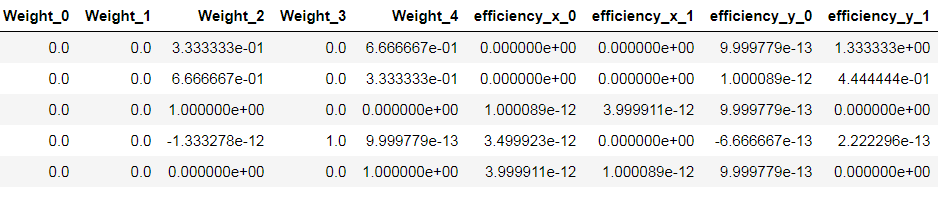该模块依据了这个公式：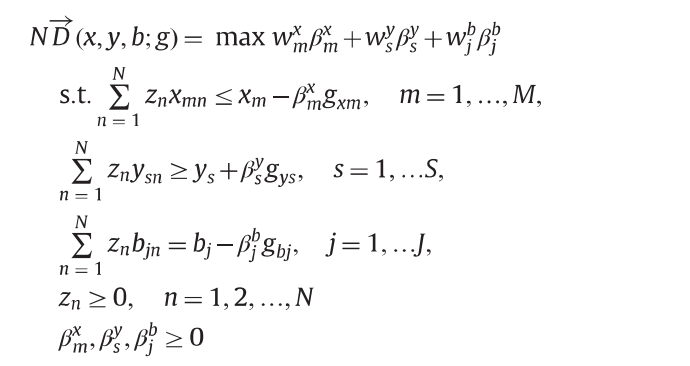文章是张宁老师13年发表在RESR上的综述，

该模块可以自行设定投入X, 产出Y, 方向向量g, 以及权重向量w

后续还得考虑把undesirable output也加入进去。

我在编程的时候发现产出扩张系数是有可能大于1的，我以前一直以为扩张系数就是无效率值，发现还是有些不一样的。

——————update——————
考虑非期望产出的NDDF
考虑强\弱处置性的非期望产出NDDF

## Cite the work

Yang, F.; Choi, Y.: Lee, H. Life-cycle data envelopment analysis to measure efficiency and cost-effectiveness of environmental regulation in China’s transport sector. Ecological indicators 2021
Choi, Y.; Yang, F.; Lee, H. On the Unbalanced Atmospheric Environmental Performance of Major Cities in China. Sustainability 2020, 12, 5391. https://doi.org/10.3390/su12135391

————————

专注于分享利用python科研的技巧，欢迎一起交流、学习、合作。

关于我的博客内容、其他相关的研究问题，有问题可以下方👇评论、或私信我~

展开全文• 关于MaxDEA 8软件求解非径向方向距离函数 (non-radial directional distance function)的说明；SuperEfficiency；Weighted；Window；Weak；Undesirable；TwoStageNetwork；RestrictedProjection；...
• DDF方向距离函数--文献文献介绍内容介绍方向距离函数DDF定义效率评估Directional calculation method(DVS)三级目录 文献介绍 文献名字是：Expected efficiency based on directional distance function in data ...

# 文献介绍

文献名字是：Expected efficiency based on directional distance function in data envelopment analysis。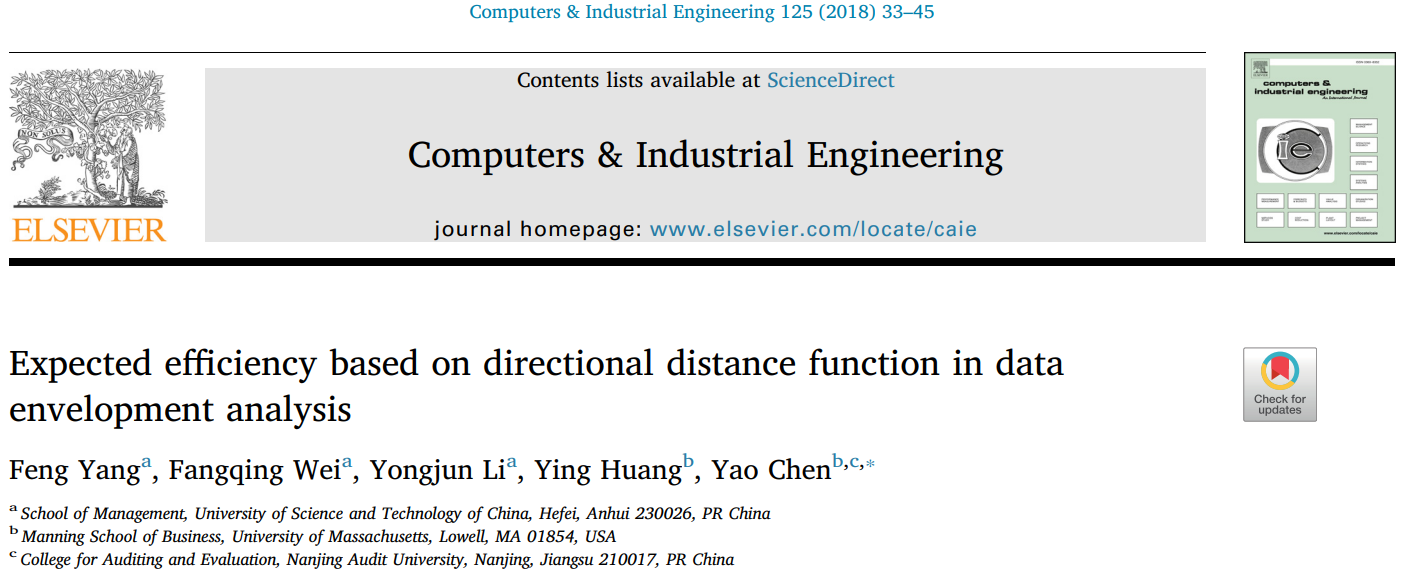首先，这篇文章是在2018年 c o m p u t e r I n d u s t r i a l E n g i n e e r i n g computer Industrial Engineering 上发表的，还有李勇军老师!!!
这篇文章偏模型，是期望DDF模型，有兴趣的朋友可以看看。

# 内容介绍

## 方向距离函数DDF定义

首先，方向距离函数。也就是 D D F DDF 函数，是一种不受径向限制的、沿预先确定的方向向量估计决策单元相对效率的方法。

比如说，有 n n D M U s DMU_s ，且投入变量与产出变量分别用矩阵表示为 X = ( x i j ) ∈ ℜ m × n X=(x_{ij})\in\Re^{m\times n} Y = ( y r j ) ∈ ℜ s × n Y=(y_{rj})\in\Re^{s\times n} 。本文假设规模报酬是可变的，即是 V R S VRS 的，那么此时的生产可能集就变成：
T = ⟮ ( x i , y r ) ∣ ∑ j λ j x i j ≤ x i , i = 1 , … , m , ∑ j λ j y r j ≥ y r , r = 1 , … , s , ∑ j λ j = 1 , λ j ≥ 0 , j = 1 , … , n ⟯ T=\lgroup(x_i,y_r)\vert\sum_{j}\lambda_jx_{ij}\leq{x_i},i=1,\dots,m,\sum_{j}\lambda_jy_{rj}\ge{}y_r,r=1,\dots,s,\sum_{j}\lambda_j=1,\lambda_{}j\ge0,j=1,\dots,n\rgroup

把方向向量记作 g = ( − g x , g y ) ≠ 0 m + s , g x ∈ R + m , g y ∈ R + s g=(-g_x,g_y)\ne0_{m+s},g_x\in{}R_{+}^{m},g_y\in{}R_{+}^{s} ，那么这个方向距离函数可以表示成：
a ⃗ T ( x , y ； g ) = s u p { β : ( x − β g x , y + β g y ) ∈ T } \vec{a}_T(x,y；g)=sup\{\beta: (x-\beta{}g_x, y+\beta{}g_y)\in{}T\}

那么，接下来，给出最初始的 D D F DDF 模型：
m a x β s . t . ∑ j = 1 n λ j x i j ≤ x i 0 − β g x , i = 1 , … , m ∑ j = 1 n λ j y r j ≥ y r 0 + β g y , r = 1 , … , s ∑ j = 1 n λ j = 1 , j = 1 , … , n λ j ≥ 0 , β ≥ 0 max \beta\\s.t.\sum_{j=1}^{n}\lambda_jx_{ij}\leq{}x_{i0}-\beta{}g_x,i=1,\dots,m \\\sum_{j=1}^{n}\lambda_{j}y_{rj}\geq{}y_{r0}+\beta{}g_y,r=1,\dots,s\\\sum_{j=1}^{n}\lambda_{j}=1,j=1,\dots,n\\\lambda_j\ge0,\beta\ge0

在上述模型中， β \beta 是用来衡量无效率的一个变量。当 β = 0 \beta=0 时候，意味着 D M U 0 DMU_{0} 是有效的。但是存在着一个问题，方向向量 g = ( − g x , g y ） g=(-g_x,g_y） 是可以任意选择的。（针对这一点，文献中用期望效率来解决。）

## 效率评估

如果按着上述公式，只看 β = 0 \beta=0 的值就进行是否有效的判断，是不合理的。因为 β = 0 \beta=0 的大小受方向向量大小的影响，若有些方向向量取得过小，可能会导致 β > 1 \beta>1

为解决这个问题，针对方向向量 g = ( − g x , g y ) , g x ∈ R + m , g y ∈ R + s , ∀ i , r g=(-g_x,g_y),g_x\in{R_{+}^{m}},g_y\in{R_{+}^{s}},\forall{i,r} ，目标函数改为：

θ 0 = 1 − 1 m ∑ i = 1 m β g x i x i 0 1 + 1 s ∑ r = 1 s β g y r y r 0 \theta_{0}=\frac{1-\frac{1}{m}\sum_{i=1}^{m}\beta{\frac{g_{xi}}{x_{i0}}}}{1+\frac{1}{s}\sum_{r=1}^{s}\beta{\frac{g_{yr}}{y_{r0}}}}

其中， β g x i x i 0 \beta{\frac{g_{xi}}{x_{i0}}} β g y r y r 0 \beta{\frac{g_{yr}}{y_{r0}}} 分别是缩小投入的比例及扩大产出的比例。

基于新的目标函数，上述模型公式可转变成为：

θ 0 = 1 − 1 m ∑ i = 1 m β g x i x i 0 1 + 1 s ∑ r = 1 s β g y r y r 0 s . t . ∑ j = 1 n λ j x i j ≤ x i 0 − β g x , i = 1 , … , m ∑ j = 1 n λ j y r j ≥ y r 0 + β g y , r = 1 , … , s ∑ j = 1 n λ j = 1 , j = 1 , … , n λ j ≥ 0 , β ≥ 0 \theta_{0}=\frac{1-\frac{1}{m}\sum_{i=1}^{m}\beta{\frac{g_{xi}}{x_{i0}}}}{1+\frac{1}{s}\sum_{r=1}^{s}\beta{\frac{g_{yr}}{y_{r0}}}}\\s.t.\sum_{j=1}^{n}\lambda_jx_{ij}\leq{}x_{i0}-\beta{}g_x,i=1,\dots,m \\\sum_{j=1}^{n}\lambda_{j}y_{rj}\geq{}y_{r0}+\beta{}g_y,r=1,\dots,s\\\sum_{j=1}^{n}\lambda_{j}=1,j=1,\dots,n\\\lambda_j\ge0,\beta\ge0

考虑到单位不变这个性质，这篇文献中是对原始数据做了归一化处理。由于在目标函数中，原始数据会作为分母进行运算，因此，处理的原则就是，数据不可以变为0。
归一化处理一般的有三种方式。这里只提及文献中所说的，令：
x ^ i j = x i j m a x j x i j , y ^ r j = y r j m a x j y r j , i = 1 , … , m ； r = 1 , … , s ； j = 1 , … , n \widehat{x}_{ij}=\frac{x_{ij}}{\mathop{max}\limits_{j}{x_{ij}}},\widehat{y}_{rj}=\frac{y_{rj}}{\mathop{max}\limits_{j}{y_{rj}}},i=1,\dots,m；r=1,\dots,s；j=1,\dots,n

由于原始数据的改变，那么对应模型公式的符号作出相应的变化：
θ 0 = 1 − 1 m ∑ i = 1 m β g x i x ^ i 0 1 + 1 s ∑ r = 1 s β g y r y ^ r 0 s . t . ∑ j = 1 n λ j x ^ i j ≤ x ^ i 0 − β g x , i = 1 , … , m ∑ j = 1 n λ j y ^ r j ≥ y ^ r 0 + β g y , r = 1 , … , s ∑ j = 1 n λ j = 1 , j = 1 , … , n λ j ≥ 0 , β ≥ 0 \theta_{0}=\frac{1-\frac{1}{m}\sum_{i=1}^{m}\beta{\frac{g_{xi}}{\widehat{x}_{i0}}}}{1+\frac{1}{s}\sum_{r=1}^{s}\beta{\frac{g_{yr}}{\widehat{y}_{r0}}}}\\s.t.\sum_{j=1}^{n}\lambda_j\widehat{x}_{ij}\leq{}\widehat{x}_{i0}-\beta{}g_x,i=1,\dots,m \\\sum_{j=1}^{n}\lambda_{j}\widehat{y}_{rj}\geq{}\widehat{y}_{r0}+\beta{}g_y,r=1,\dots,s\\\sum_{j=1}^{n}\lambda_{j}=1,j=1,\dots,n\\\lambda_j\ge0,\beta\ge0

文献中还特别提及，此时，不管方向向量取值多少，都不会改变目标函数的值。（若有兴趣看证明，可自行查阅文献，打公式太累了）

## Directional calculation method(DVS)

这块知识，涉及到了辛钦大数定律的知识。

文献中简单介绍了DVS的内容：
U ^ \widehat{U} 作为欧几里得空间非负象限中的所有单位向量的集合，是以圆点为中心，半径为1的向量集合，是无限集合。并且把 E ^ \widehat{E} 作为从 U ^ \widehat{U} 中均匀抽取的集合，这个 E ^ \widehat{E} 就被叫做方向向量集合，是有限集合。

只要样本量足够大， E ^ \widehat{E} 的集合接近 U ^ \widehat{U}

核心思想就是：方向向量均匀取值得足够多，也就是方向向量旋转角度足够小，计算出来的效率值就接近真实期望值。

### 具体算法

这里只介绍三位情况：
对于三维情况，也就是两个投入、一个产出时， U ^ 3 = { ( v 1 , v 2 , v 3 ) ∣ 0 ≤ v 1 , v 2 , v 3 ≤ 1 } \widehat{U}^{3}=\lbrace{(v_1,v_2,v_3)|0\leq{}v_1,v_2,v_3\leq{}1}\rbrace ，这是一个三维空间上非负象限里以圆点为中心，半径为1的球体部分。如图：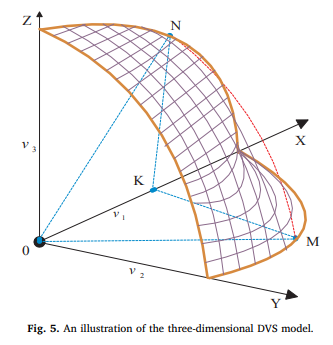step1: 令 v 1 = sin ⁡ ( d ∗ ϕ ) , d = 0 , 1 , 2 , … , π / 2 ϕ v_1=\sin(d*\phi),d=0,1,2,\dots,\pi/2\phi ，其中 ϕ \phi 是旋转区间角度。
step2:当 d = 0 d=0 时，那么令 v 1 = 0 v_1=0 。如果 ( l ∗ ϕ ) / 1 − ( sin ⁡ 2 ( d ∗ ϕ ) ) > π / 2 (l*\phi)/\sqrt{1-(\sin^2(d*\phi))}>\pi/2 ，那么 v 2 = 1 − v 1 2 v_2=\sqrt{1-v_1^{2}} 并且 v 3 = 0 v_3=0 ；或者说如果 v 1 2 + ( 1 − sin ⁡ 2 ( d ∗ ϕ ) ∗ sin ⁡ ( l ∗ ϕ / 1 − sin ⁡ 2 ( d ∗ ϕ ) ) ) 2 ≤ 1 v_1^{2}+(\sqrt{1-\sin^2(d*\phi)}*\sin(l*\phi/\sqrt{1-\sin^2(d*\phi)}))^2\le1 ，那么令 v 2 = 1 − sin ⁡ 2 ( d ∗ ϕ ) ∗ sin ⁡ ( 2 sin ⁡ − 1 ( sin ⁡ ( l ∗ ϕ / 2 ) / 1 − sin ⁡ 2 ( d ∗ ϕ ) ) ) v_2=\sqrt{1-\sin^2(d*\phi)}*\sin(2\sin^{-1}(\sin{(l*\phi/2})/\sqrt{1-\sin^2(d*\phi)})) ，并且如果 1 − v 1 2 − v 2 2 > 0 1-v_1^2-v_2^2>0 ，那么 v 3 = 1 − v 1 2 − v 2 2 v_3=\sqrt{1-v_1^2-v_2^2} 。当 l = 0 , 1 , 2 , … , π / 2 ϕ l=0,1,2,\dots,\pi/2\phi ，遍历step2。
step3:当 d = 0 , 1 , 2 , … , π / 2 ϕ d=0,1,2,\dots,\pi/2\phi 遍历步骤2。

## three-dimension 例子

这里，拿出文献中的example1进行说明。

首先，数据为两个 投入一个产出的69个DMUs。（文献中没有给出原始数据，但是由于这篇文章主要阐述的模型，所以如果真想实现的话，可以自行搜索数据）

将数据放入模型中，作者说总共得到了20933个方向向量，但是我个人复现的时候，却有29400左右个，很迷惑。

若有朋友对这篇文献有兴趣，欢迎一起讨论。

展开全文python
• 将DEA方法应用于技术效率测算，与...非径向 DEA方法运用定向技术距离函数，投影方向具有一般性，而且同时考虑投入和产出两个方面，将其应用于技术效率测算对技术效率测算。理论的完善、应用领域的拓展具有重要意义。
• ## 高斯径向基函数(RBF)神经网络

万次阅读 多人点赞 2019-04-03 00:53:52
高斯径向函数(RBF)神经网络 牛顿插值法-知乎 ...说径向基网络之前，先聊下径向函数径向函数（英语：radial basis function，缩写为RBF）是一个取值仅依赖于到原点距离的实值函数，即 ϕ(x...

# 高斯径向基函数(RBF)神经网络

牛顿插值法-知乎
泰勒公式
径向基函数-wiki
径向基网络之bp训练
RBF网络逼近能力及其算法
线性/非线性，使用”多项式“逼近非线性，通过调节超参数来改善多项式参数进一步拟合真实非线性。

# 径向基函数

说径向基网络之前，先聊下径向基函数径向基函数（英语：radial basis function，缩写为RBF）是一个取值仅依赖于到原点距离的实值函数，即 ϕ ( x ) = ϕ ( ∥ x ∥ ) {\displaystyle \phi (\mathbf {x} )=\phi (\|\mathbf {x} \|)} 。此外，也可以按到某一中心点c的距离来定义， 即 ϕ ( x , c ) = ϕ ( ∥ x − c ∥ ) {\displaystyle \phi (\mathbf {x} ,\mathbf {c} )=\phi (\|\mathbf {x} -\mathbf {c} \|)} 。任一满足 ϕ ( x ) = ϕ ( ∥ x ∥ ) {\displaystyle \phi (\mathbf {x} )=\phi (\|\mathbf {x} \|)} 的函数都可称作径向函数。其中，范数一般为欧几里得距离，不过亦可使用其他距离函数。

可以用于许多径向基函数的和来逼近某一给定的函数。这一逼近的过程可看作是一个简单的神经网络(rbf网络中的每个隐单元看作一个径向基函数，然后再线性加权结合逼近)。此外在机器学习中，径向基函数还被用作支持向量机的核函数。

看了好多博客基本都没有谈到为什么径向基函数可以逼近函数，说数谈的也说的不清楚，强硬开始径向基网络的分析，以下是我的一些见解
既然是逼近函数，那么建议读者先简单通过顶部的知乎链接了解下逼近这个概念和多变量插值问题。

为什么径向基函数可以逼近给定函数呢？ 换句话说也就是使用径向基函数解决多变量插值问题，从几何意义上看，相当于根据稀疏的给定样本数据点恢复一个连续的超曲面，在给定点处曲面的值要满足样本值。先给出基于径向基函数的插值函数如下：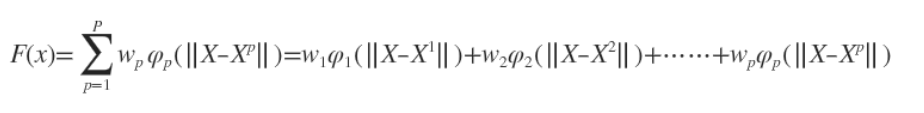可以看出插值函数就是由p个径向基函数和其权值构成(p为给定的样本数)，那么也就意味着逼近的这个超曲面上任何一点可以由对应的基函数值得出，具体实例看下图(搬运)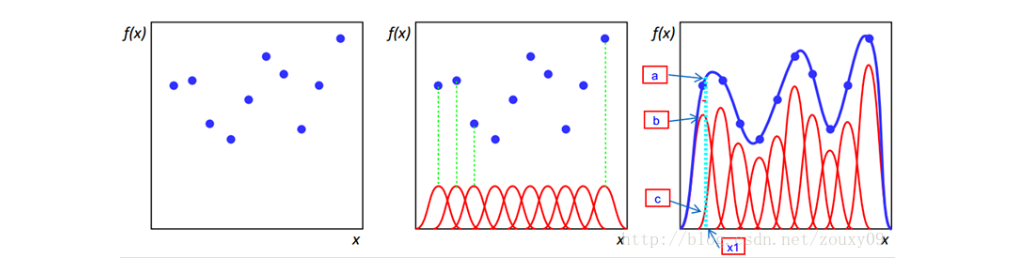第一张图即给出二维平面中的n个样本点，然后构建n个基函数，如图二中红色的曲线，假设采用高斯径向基函数，其对应的曲线即高斯分布曲线，图三中的蓝色曲线即真正曲线，多维情况下即为超曲面。而我们高斯径向基函数要做的就是用这n个高斯曲线去拟合这条蓝色曲线。可以看出图三中的红色曲线和图二中的曲线不同，这是由图二的曲线乘以一个权值得到的，这也就对应了上面说到的插值函数就是由p个径向基函数和其权值构成(p为给定的样本数)。例如对x1，其真实值为f(x1)，即图三中的a点，而与高斯曲线相交于b、c两点，高斯径向基函数拟合的结果就是b和c的纵坐标之和，f(x1)-b-c就是误差，我们要做的就是优化权值参数或者选取其他径向基函数来尽可能还原蓝色曲线。

# RBF网络

BF网络能够逼近任意的非线性函数，可以处理系统内的难以解析的规律性，具有良好的泛化能力，并有很快的学习收敛速度，已成功应用于非线性函数逼近、时间序列分析、数据分类、模式识别、信息处理、图像处理、系统建模、控制和故障诊断等。

简单说明一下为什么RBF网络学习收敛得比较快。当网络的一个或多个可调参数（权值或阈值）对任何一个输出都有影响时，这样的网络称为全局逼近网络。由于对于每次输入，网络上的每一个权值都要调整(例如传统的多项式插值法)，从而导致全局逼近网络的学习速度很慢。BP网络就是一个典型的例子。

如果对于输入空间的某个局部区域只有少数几个连接权值影响输出，则该网络称为局部逼近网络。常见的局部逼近网络有RBF网络、小脑模型（CMAC）网络、B样条网络等。

明白RBF如何逼近一个给定函数后，RBF网络就是用网络来实现上述思路。
径向基函数网络通常有三层：输入层、隐藏层和一个非线性激活函数和线性径向基神经网络输出层。输入可以被建模为实数向量。输出是输入向量的一个标量函数。rbf简单网络模型如下：RBF网络的基本思想是：用RBF作为隐单元的“基”构成隐含层空间，这样就可以将输入矢量直接映射到隐空间，而不需要通过权连接。当RBF的中心点确定以后，这种映射关系也就确定了。而隐含层空间到输出空间的映射是线性的，即网络的输出是隐单元输出的线性加权和，此处的权即为网络可调参数。其中，隐含层的作用是把向量从低维度的p映射到高维度的h，这样低维度线性不可分的情况到高维度就可以变得线性可分了，主要就是核函数的思想。这样，网络由输入到输出的映射是非线性的，而网络输出对可调参数而言却又是线性的。网络的权就可由线性方程组直接解出，从而大大加快学习速度并避免局部极小问题。

# RBF网络训练方法

我们由RBF函数过渡到RBF网络，接下来我们研究如何搭建一个RBF网络，由上述内容我们可以RBF网络无非3层，而输入层是无法优化的，只有隐藏层和输出层了，因此RBF网络训练就是对两组网络参数的学习：
1.隐层节点中心、RBF宽度、以及隐层节点数
2.隐层到输出层连接权值

1、方法一：

通过非监督方法得到径向基函数的中心和方差，通过监督方法（最小均方误差）得到隐含层到输出层的权值。具体如下：

（1）在训练样本集中随机选择h个样本作为h个径向基函数的中心。更好的方法是通过聚类，例如K-means聚类得到h个聚类中心，将这些聚类中心当成径向基函数的h个中心。

（2）RBF神经网络的基函数为高斯函数时，方差可由下式求解：式中cmax 为所选取中心之间的最大距离，h是隐层节点的个数。扩展常数这么计算是为了避免径向基函数太尖或太平。

（3）隐含层至输出层之间神经元的连接权值可以用最小均方误差LMS直接计算得到，计算公式如下：（计算伪逆）（d是我们期待的输出值）2、方法二：

采用监督学习算法对网络所有的参数（径向基函数的中心、方差和隐含层到输出层的权值）进行训练。主要是对代价函数（均方误差）进行梯度下降，然后修正每个参数。具体如下：

（1）随机初始化径向基函数的中心、方差和隐含层到输出层的权值。当然了，也可以选用方法一中的（1）来初始化径向基函数的中心。

（2）通过梯度下降来对网络中的三种参数都进行监督训练优化。代价函数是网络输出和期望输出的均方误差：然后每次迭代，在误差梯度的负方向已一定的学习率调整参数。

# RBF网络与BP网络对比

1、局部逼近与全局逼近：

BP神经网络的隐节点采用输入向量与权向量的内积作为激活函数的自变量，而激活函数采用Sigmoid函数。各调参数对BP网络的输出具有同等地位的影响，因此BP神经网络是对非线性映射的全局逼近，每当有新的样本出现时，都要重新计算参数，训练很慢。

RBF神经网络的隐节点采用输入向量与中心向量的距离（如欧式距离）作为函数的自变量，并使用径向基函数（如Gaussian函数）作为激活函数。神经元的输入离径向基函数中心越远，神经元的激活程度就越低（高斯函数）。RBF网络的输出与部分调参数有关，譬如，一个wij值只影响一个yi的输出（参考上面的径向函数3张图，目标函数的逼近只靠最近的几个径向函数来实现，而距离远的径向函数不起作用，隐节点的这一特性常被称为“局部特性”。），RBF神经网络因此具有“局部逼近”特性。

简单说，假设输入向量的取值范围是[0,1]，那么全局逼近就是有n个函数，每个函数的自变量输入范围是[0,1]，每输入一个变量，会得到n个值，这n个值相加之和就是逼近的真实值，也就是说用全部函数来逼近目标函数。而局部逼近也是n个函数，但是每个函数的自变量是1/n，那么输入一个变量，只会得到一个值，这个值就是逼近的真实值，也就是说用一个函数来逼近目标函数，实际是数个函数，所以说是局部逼近。(以上例子仅为了通俗对比二者而举)

2、中间层数的区别
BP神经网络可以有多个隐含层，但是RBF只有一个隐含层。

3、训练速度的区别
使用RBF的训练速度快，一方面是因为隐含层较少，另一方面，局部逼近可以简化计算量。对于一个输入x，只有部分神经元会有响应，其他的都近似为0，对应的w就不用调参了。

4、Poggio和Girosi已经证明，RBF网络是连续函数的最佳逼近，而BP网络不是。

# RBF网络和SVM对比

SVM等如果使用核函数的技巧的话，不太适应于大样本和大的特征数的情况(因为SVM间隔最大化是一个二次规划问题，求解将涉及m阶矩阵的计算(m为样本的个数), 因此SVM不适用于超大数据集)，因此提出了RBF。

另外，SVM中的高斯核函数可以看作与每一个输入点的距离，而RBF神经网络对输入点做了一个聚类。RBF神经网络用高斯核函数时,其数据中心C可以是训练样本中的抽样，此时与svm的高斯核函数是完全等价的，也可以是训练样本集的多个聚类中心，所以他们都是需要选择数据中心的，只不过SVM使用高斯核函数时，这里的数据中心都是训练样本本身而已。# 高斯核函数为什么可以将低维映射到高维

相信大部分人都听过核函数可以将低维数据映射到高维中，其实准确说核函数只是给出了数据在低维下计算高维内积的方法。

对于高斯核为什么可以将数据映射到无穷多维，我们可以从泰勒展开式的角度来解释，

首先我们要清楚，SVM中，对于维度的计算，我们可以用内积的形式，假设函数：
κ ( x 1 , x 2 ) = ( 1 , x 1 x 2 , x 2 ) \kappa \left( x_{1}, x_{2} \right) = (1, x_{1}x_{2}, x^{2} ) 表示一个简单的从二维映射到三维。

则在SVM的计算中，可以表示为：
κ ( x 1 , x 2 ) = 1 + x 1 x 2 + x 2 \kappa \left( x_{1}, x_{2} \right) = 1+x_{1}x_{2}+ x^{2}

再来看 e x e^{x} 泰勒展开式(具体泰勒展开公式推导见顶部知乎链接)：
e x ≈ 1 + x + x 2 2 ! + x 3 3 ! + . . . + x n n ! e^{x} \approx 1 + x + \frac{x^{2}}{2!} + \frac{x^{3}}{3!} + ... + \frac{x^{n}}{n!}

所以这个无穷多项的式子正是对于 e x e^{x} 的近似， e x e^{x} 所对应的映射：
κ ( x ) = ( 1 , x , x 2 2 ! , x 3 3 ! , . . . , x n n ! ) \kappa \left( x \right) = \left( 1, x, \frac{x^{2} }{2!}, \frac{x^{3} }{3!}, ..., \frac{x^{n} }{n!} \right)

再来看高斯核：
κ ( x 1 , x 2 ) = e ( − ∣ ∣ x 1 − x 2 ∣ ∣ 2 2 σ 2 ) \kappa \left( x_{1} , x_{2} \right) = e^{\left(- \frac{\left||x_{1} - x_{2} \right|| ^{2} }{2\sigma ^{2} } \right) }

将泰勒展开式带入高斯核，我们得到了一个无穷维度的映射
κ ( x 1 , x 2 ) = 1 + ( − ∣ ∣ x 1 − x 2 ∣ ∣ 2 2 σ 2 ) + ( − ∣ ∣ x 1 − x 2 ∣ ∣ 2 2 σ 2 ) 2 2 ! + . . . + ( − ∣ ∣ x 1 − x 2 ∣ ∣ 2 2 σ 2 ) 3 3 ! + . . . + ( − ∣ ∣ x 1 − x 2 ∣ ∣ 2 2 σ 2 ) n n ! \kappa \left( x_{1} , x_{2} \right) = 1 + \left(- \frac{\left||x_{1} - x_{2} \right|| ^{2} }{2\sigma ^{2} } \right) + \frac{(-\frac{\left||x_{1} - x_{2} \right|| ^{2} }{2\sigma ^{2} })^{2} }{2!} + ... + \frac{(-\frac{\left||x_{1} - x_{2} \right|| ^{2} }{2\sigma ^{2} })^{3} }{3!} + ... + \frac{(-\frac{\left||x_{1} - x_{2} \right|| ^{2} }{2\sigma ^{2} })^{n} }{n!}
即：当n趋于正无穷时，对于 x 1 x_{1} x 2 x_{2} 的内积形式符合在SVM中无穷维度下的内积计算，即高斯核将数据映射到无穷高的维度。

# 前馈网络、递归网络和反馈网络

前馈网络一般指前馈神经网络或前馈型神经网络。它是一种最简单的神经网络，各神经元分层排列。每个神经元只与前一层的神经元相连。接收前一层的输出，并输出给下一层，各层间没有反馈。包括：BP神经网络、RBF神经网络等。

递归神经网络（RNN）是两种人工神经网络的总称。一种是时间递归神经网络（recurrent neural network），又名循环神经网络，包括RNN、LSTM、GRU等；另一种是结构递归神经网络（recursive neural network）。

反馈网络(Recurrent Network)，又称自联想记忆网络，其目的是为了设计一个网络，储存一组平衡点，使得当给网络一组初始值时，网络通过自行运行而最终收敛到这个设计的平衡点上。包括CHNN、DHNN等。

展开全文RBF 机器学习
• 径向分布函数g(r)代表了球壳内的平均数密度为离中心分子距离为r，体积为 的球壳内的瞬时分子数。具体参见李如生，《平衡和平衡统计力学》科学出版社：1995 CODE: SUBROUTINE GR(NSWITCH) IMPLICIT DOUBLE ...
• ## RBF（径向基函数）神经网络

万次阅读 多人点赞 2017-07-06 11:01:32
2、得到距离之后，将距离代入径向函数，得到一个数值。数值再与后边的权值相乘再求总和，就得到了相应输入的输出。 3、在训练网络之前，需要确定中心点的个数，和中心点的位置。以及求出隐藏层各径向函数的方差...机器学习 神经网络
• 介绍核函数的不同类型、核函数在不同数据集上的表现，在rbf上表现最好。以及核函数的缺点，提醒我们需要将数据进行统一量纲处理。机器学习 sklearn python
• Deep Learning 深度学习...概念于2006年由Hinton提出，基于深信度网DBN提出的监督的贪心逐层训练算法，为解决深层结构相关的优化难题带来希望，随后提出多层自动编码器深层结构。此外Lecun等人提出的卷积神经网络...
• 径向函数（RBF）神经网络 RBF网络能够逼近任意的线性函数，可以处理系统内的难以解析的规律性，具有良好的泛化能力，并有很快的学习收敛速度，已成功应用于线性函数逼近、时间序列分析、数据分类、模式...
• ## 高斯核函数

万次阅读 多人点赞 2019-10-24 18:59:14
高斯核函数 (Gaussian kernel)，也称径向基 (RBF) 函数，就是某种沿径向对称的标量函数，用于将有限维数据映射到高维空间。通常定义为空间中任意一点到某一中心点之间的欧式距离的单调函数，可记作,其作用往往是局部...
•   由于之前做了很多核方法相关...首先，再对核方法的思想进行描述，核函数的思想是一个伟大的想法，它工作简练巧妙的映射，解决了高维空间中数据量庞大的问题，在机器学习中是对算法进行线性改进的利器。如下，...机器学习
• 通常定义为空间中任一点x到某一中心xc之间欧氏距离的单调函数, 可记作 k(||x-xc||), 其作用往往是局部的 , 即当x远离xc时函数取值很小。最常用的径向函数是高斯核函数 ,形式为k(||x-xc||)=exp{- ||x-xc||^2/(2*σ...
• 本文首先介绍Levenshtein 距离（编辑距离）算法，以其为例对比二元函数一节泰勒公式与动态规划状态转移函数的相似点，为有高等数学基础的读者提供一种对动态规划新的理解方式。这种理解可拓展到所有双字符串动态规划...动态规划 python
• 研究结果表明:深孔注水区域内的煤体处于卸载破坏区,该区域内的注水过程属于渗流层流,沿钻孔径向方向上的水力压头具有余误差函数特性,其渗流边界的扩展速度及钻孔注水流量均随时间逐渐减小;而沿钻孔深度方向上,随着煤...
• SVM中为什么需要核函数？ 核密度估计的核又是什么？ KPCA中的核的作用是什么？ 深度学习CNN中的卷积核？核和过滤有又什么关系? 核密度估计又是什么？ 核函数和激活函数什么关系？ Linux内核叫Kernel? Jupyter...数据挖掘 机器学习 svm
• Python3：《机器学习实战》之支持向量机（4）核函数及其实现 转载请注明作者和出处：http://blog.csdn.net/u011475210 代码地址：https://github.com/WordZzzz/ML/tree/master/Ch06 操作系统：WINDOWS 10 软件版本：...机器学习 SVM 径向基核函数
• 在OpenCV中提供了各种特征检测函数和特征提取函数，其中包括有边缘检测（canny），角点检测等，如：Harris角点、ShiTomasi角点、亚像素级角点、SURF角点、Star关键点、FAST关键点、Lepetit关键点等等。下面将对特征...opencv
• 1，线性逼近 1.1，基本原理 ...若状态空间的维数很大，如围棋（个状态空间），此时精确获取各种和几乎不可能的，因为既没有足够的内存也没有足够的计算能力，这时候需要找到近似的函数，利用函数逼近的方...机器学习
• 本篇文章为转载，真的觉得这篇文章写的详细易懂，推导也写的...另外还有一篇专门写激活函数的文章：https://blog.csdn.net/tyhj_sf/article/details/79932893 首发于腾讯技术 已关注写文章 AI从入门到放弃...
• 接下来这节内容，选材来自昨天学习交流群中一位朋友，提出了有关激活函数的问题。我在收集了一下相关内容，在此整理集合对比激活函数的缺点和不足。 什么是激活函数 文章主要从激活函数概念，数学形式分析，Python...神经网络 python 机器学习
• 点扩展函数(point spread functionPSF)描述了成像系统对点源或点对象的响应。PSF更一般的术语是一个系统的脉冲响应（impulse response），PSF是聚焦光学系统的脉冲响应。PSF在许多情况下可以被当作是图像中表示一个...
• 关于机器学习Linear classification(线性分类问题)Linear Regression (线性回归问题)Radial Basis Function (RBF) (径向函数)Multi-layer Perceotron (多层感知机)& 反向传播 Linear classification(线性分类...
• 影响拍摄质量最重要的因素是镜头的分辨率和反差。反差大小可以通过仪器很容易测量，而分辨率就不那么容易了！...由数学证明可知，任何周期性图形都可以分解成亮度按正弦变化的图形的叠加，而任何周期图形
• 关于理论部分，参看其他书本，这里演示支持向量机在线性数据集上不可分，经过核函数变换后变成线性可分。 一、使用步骤 画图显示 代码如下（示例）： from sklearn.svm import SVC import matplotlib.pyplot as ...机器学习 python
•数据挖掘
• 在投影矩阵前加上个畸变模型大小为3*3的Slamda，相当于在坐标映射前加了x-y方向的放缩系数。 lamda通过一个与中心点距离相关的多项式拟合。 将畸变的投影矩阵定义为Q，得到Q矩阵的映射关系： 像素与三维...计算机视觉 人工智能...